Ch 6. Entropy Multimedia Engineering Thermodynamics Entropy Tds Relations EntropyChange IsentropicProcess IsentropicEfficiency EntropyBalance (1) EntropyBalance (2) ReversibleWork
 Chapter 1. Basics 2. Pure Substances 3. First Law 4. Energy Analysis 5. Second Law 6. Entropy 7. Exergy Analysis 8. Gas Power Cyc 9. Brayton Cycle 10. Rankine Cycle Appendix Basic Math Units Thermo Tables Search eBooks Dynamics Fluids Math Mechanics Statics Thermodynamics Author(s): Meirong Huang Kurt Gramoll ©Kurt GramollTHERMODYNAMICS - THEORY Isentropic Processes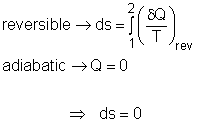A Reversible and Adiabatic Process is also an Isentropic Process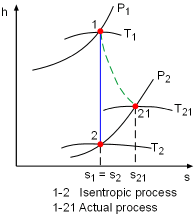An Isentropic Process and an Actual Process on a h-s Diagram The term "isentropic" means constant entropy. A process during which the entropy remains constant is called an isentropic process, which is characterized by       ΔS = 0 or s1 = s2 for a process 1-2 If a process is both reversible and adiabatic, then it is an isentropic process. An isentropic process is an idealization of an actual process, and serves as a limiting case for an actual process. Isentropic Processes for Ideal Gases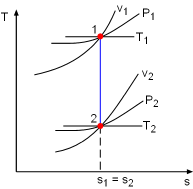An Isentropic Process of Ideal Gases on a T-s Diagram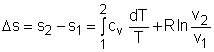(1) and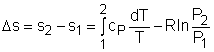(2) By setting Δs to 0 in the above equations, the relations for an ideal gas which undergoes an isentropic process can be obtained. Setting equation (1) to zero gives,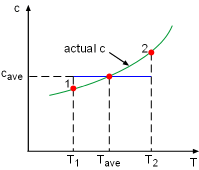Constant Specific Heat Used in Small Temperature Interval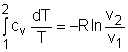If the constant-specific-heats assumption is valid, the above equation can be integrated and rearranged to give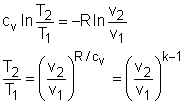where       k = specific heat ratio, k = cP/cv and R = cP - cv The second relation can be obtained by setting equation (2) to zero.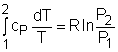Also, if the constant-specific-heats assumption is valid, the above equation becomes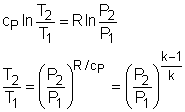The third relation can be obtained by combining the first and the second relations. That is,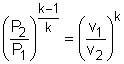The three relations of an isentropic process for ideal gases with constant specific heats in compact form are       Tvk-1 = constant       TP(1-k)/k = constant       Pvk = constant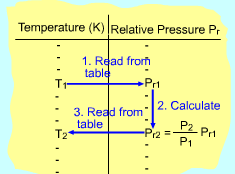Using Pr Data to Determine Final Temperature during an Isentropic Process If the constant-specific-heats assumption is not valid, the entropy change of ideal gases during a process 1-2 is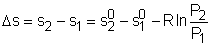Setting the above equation to zero and rearranging, one obtains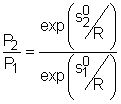If exp(s0/R) is defined as the relative pressure Pr, then the above equation becomes       (P2/P1)s = constant = Pr2/Pr1 Values of relative pressure are tabulated against temperature in tables.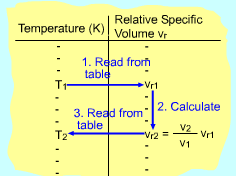Using vr Data to Determine Final Temperature during an Isentropic Process In an automotive engine, the ratio v2/ v1 is used instead of the ratio P2/ P1. The ideal-gas relation gives       v2/ v1 = T2P1/T1P2 Replacing P2/P1 by Pr2/Pr1 in the above equation gives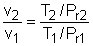T/Pr is defined as relative specific volume vr and its value is also tabulated with temperature. Thus,       (v2/v1)s = constant = vr2/ vr1 Isentropic Processes for an Incompressible Fluid or Solid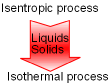Isentropic Process of an Incompressible Substance is also Isothermal The entropy change of incompressible fluids or solids during a process 1-2 with constant specific heats is       Δs = caveln(T2/T1) Setting Δs to zero gives       T2 = T1 That is, an isentropic process of an incompressible substance is also isothermal.